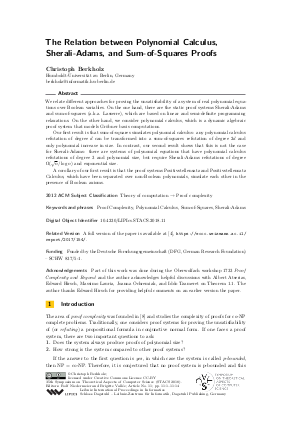Document# The Relation between Polynomial Calculus, Sherali-Adams, and Sum-of-Squares Proofs

### Author Christoph Berkholz## File

LIPIcs.STACS.2018.11.pdf
• Filesize: 0.5 MB
• 14 pages

## Cite As

Christoph Berkholz. The Relation between Polynomial Calculus, Sherali-Adams, and Sum-of-Squares Proofs. In 35th Symposium on Theoretical Aspects of Computer Science (STACS 2018). Leibniz International Proceedings in Informatics (LIPIcs), Volume 96, pp. 11:1-11:14, Schloss Dagstuhl - Leibniz-Zentrum für Informatik (2018)
https://doi.org/10.4230/LIPIcs.STACS.2018.11

## Abstract

We relate different approaches for proving the unsatisfiability of a system of real polynomial equations over Boolean variables. On the one hand, there are the static proof systems Sherali-Adams and sum-of-squares (a.k.a. Lasserre), which are based on linear and semi-definite programming relaxations. On the other hand, we consider polynomial calculus, which is a dynamic algebraic proof system that models Gröbner basis computations. Our first result is that sum-of-squares simulates polynomial calculus: any polynomial calculus refutation of degree d can be transformed into a sum-of-squares refutation of degree 2d and only polynomial increase in size. In contrast, our second result shows that this is not the case for Sherali-Adams: there are systems of polynomial equations that have polynomial calculus refutations of degree 3 and polynomial size, but require Sherali-Adams refutations of large degree and exponential size. A corollary of our first result is that the proof systems Positivstellensatz and Positivstellensatz Calculus, which have been separated over non-Boolean polynomials, simulate each other in the presence of Boolean axioms.
##### Keywords
• Proof Complexity
• Polynomial Calculus
• Sum-of-Squares

## Metrics

• Access Statistics
• Total Accesses (updated on a weekly basis)
0

## References

1. Michael Alekhnovich, Eli Ben-Sasson, Alexander A. Razborov, and Avi Wigderson. Space complexity in propositional calculus. SIAM J. Comput., 31(4):1184-1211, 2002. URL: http://dx.doi.org/10.1137/S0097539700366735.
2. Albert Atserias, Massimo Lauria, and Jakob Nordström. Narrow proofs may be maximally long. ACM Trans. Comput. Log., 17(3):19:1-19:30, 2016. URL: http://dx.doi.org/10.1145/2898435.
3. Paul Beame, Russell Impagliazzo, Jan Krajíček, Toniann Pitassi, and Pavel Pudlák. Lower bounds on hilbert’s nullstellensatz and propositional proofs. Proceedings of the London Mathematical Society, s3-73(1):1-26, 1996. URL: http://dx.doi.org/10.1112/plms/s3-73.1.1.
4. Christoph Berkholz. The relation between polynomial calculus, sherali-adams, and sum-of-squares proofs. Electronic Colloquium on Computational Complexity (ECCC), 24:154, 2017. URL: https://eccc.weizmann.ac.il/report/2017/154.
5. W. Dale Brownawell. Bounds for the degrees in the nullstellensatz. Annals of Mathematics, 126(3):577-591, 1987. URL: http://www.jstor.org/stable/1971361.
6. Josh Buresh-Oppenheim, Matthew Clegg, Russell Impagliazzo, and Toniann Pitassi. Homogenization and the polynomial calculus. Computational Complexity, 11(3-4):91-108, 2002. URL: http://dx.doi.org/10.1007/s00037-002-0171-6.
7. M. Clegg, J. Edmonds, and R. Impagliazzo. Using the Groebner basis algorithm to find proofs of unsatisfiability. In Proceedings of the 28th annual ACM symposium on Theory of computing, pages 174-183, 1996.8. Stephen A. Cook and Robert Reckhow. The relative efficiency of propositional proof systems. Journal of Symbolic Logic, 44(1):36-50, 1979.9. Stefan S. Dantchev, Barnaby Martin, and Mark Nicholas Charles Rhodes. Tight rank lower bounds for the sherali-adams proof system. Theor. Comput. Sci., 410(21-23):2054-2063, 2009. URL: http://dx.doi.org/10.1016/j.tcs.2009.01.002.
10. John R. Gilbert and Robert Endre Tarjan. Variations of a pebble game on graphs. Technical Report STAN-CS-78-661, Stanford University, 1978. Available at URL: http://infolab.stanford.edu/TR/CS-TR-78-661.html.
11. Dima Grigoriev, Edward A. Hirsch, and Dmitrii V. Pasechnik. Complexity of semi-algebraic proofs. Moscow Mathematical Journal, 2(4):647-679, 2002. URL: http://www.ams.org/distribution/mmj/vol2-4-2002/abst2-4-2002.html.
12. Dima Grigoriev and Nicolai Vorobjov. Complexity of null- and positivstellensatz proofs. Annals of Pure and Applied Logic, 113(1–3):153-160, 2001.13. Russell Impagliazzo, Pavel Pudlák, and Jirí Sgall. Lower bounds for the polynomial calculus and the gröbner basis algorithm. Computational Complexity, 8(2):127-144, 1999. URL: http://dx.doi.org/10.1007/s000370050024.
14. J. L. Krivine. Anneaux préordonnés. Journal d'Analyse Mathématique, 12(1):307-326, Dec 1964. URL: http://dx.doi.org/10.1007/BF02807438.
15. Jean B. Lasserre. Global optimization with polynomials and the problem of moments. SIAM Journal on Optimization, 11(3):796-817, 2001. URL: http://dx.doi.org/10.1137/S1052623400366802.
16. Massimo Lauria and Jakob Nordström. Tight size-degree bounds for sums-of-squares proofs. Computational Complexity, 26(4):911-948, 2017. URL: http://dx.doi.org/10.1007/s00037-017-0152-4.
17. H. Lombardi, N. Mnev, and M.-F. Roy. The positivstellensatz and small deduction rules for systems of inequalities. Math. Nachr., 181:245-259, 1996.18. László Lovász and Alexander Schrijver. Cones of matrices and set-functions and 0-1 optimization. SIAM Journal on Optimization, 1(2):166-190, 1991. URL: http://dx.doi.org/10.1137/0801013.
19. Jakob Nordström. Pebble games, proof complexity, and time-space trade-offs. Logical Methods in Computer Science, 9(3), 2013. URL: http://dx.doi.org/10.2168/LMCS-9(3:15)2013.
20. P. Parrilo. Structured Semidefinite Programs and Semialgebraic Geometry Methods in Robustness and Optimization. PhD thesis, California Institute of Technology, 2000.21. Mihai Putinar. Positive polynomials on compact semi-algebraic sets. Indiana University Mathematics Journal, 42(3):969-984, 1993. URL: http://www.jstor.org/stable/24897130.
22. H. D. Sherali and W. P. Adams. A hierarchy of relaxations between the continuous and convex hull representations for zero-one programming problems. SIAM Journal on Discrete Mathematics, 3(3):411-430, 1990.23. Gilbert Stengle. A nullstellensatz and a positivstellensatz in semialgebraic geometry. Mathematische Annalen, 207(2):87-97, Jun 1974. URL: http://dx.doi.org/10.1007/BF01362149.
X

Feedback for Dagstuhl Publishing